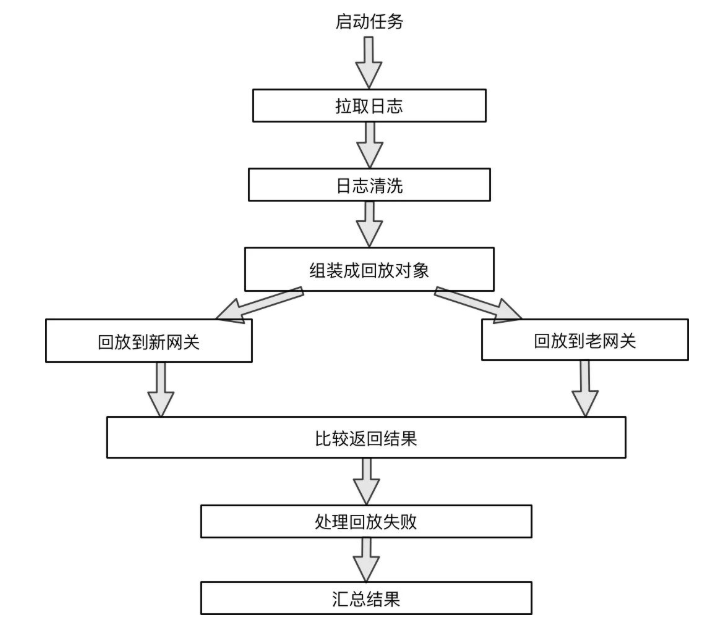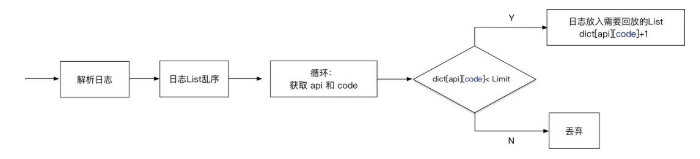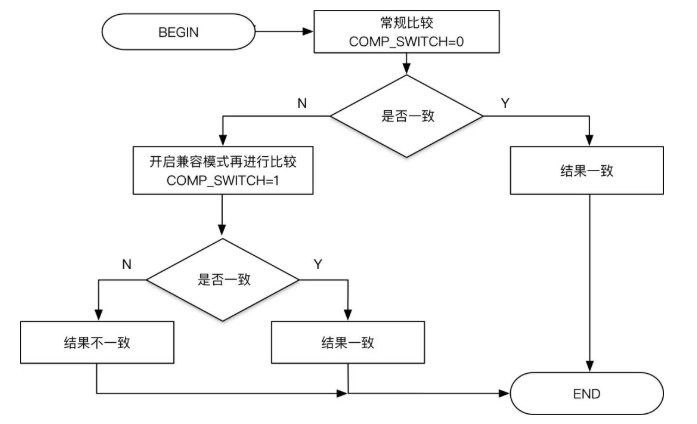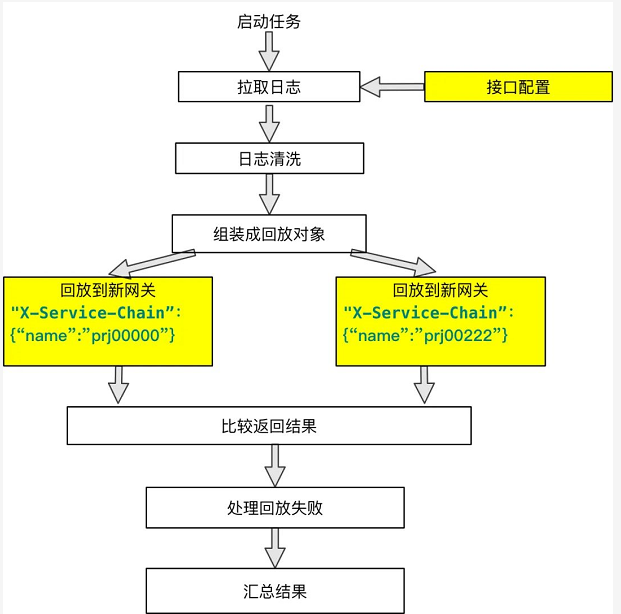# 二、系统设计## 2.1 日志拉取和清洗

1. 过滤。有一些接口是不需要迁移到新网关的，进行过滤，减少对最后统计结果的干扰。另外，录制回放运行时也会请求网关，需要过滤掉这部分人工流量。
2. 采样。虽然是测试环境，一天的数据量也有 10W+ ，全部处理时耗较长；同时，初期会有大量重复问题爆出，增大排查成本。根据二八定律以及实际的观察，小部分接口贡献了大部分流量，考虑每个接口雨露均沾和数据的多样性，选择接口+场景作为采样纬度，返回结果中的不同 code 认为是不同的场景，这样除了正常请求，也考虑到异常请求的回放。## 2.2 进行回放

``````    p = multiprocessing.Pool(8)
``````
``````    while line:
``````
``````        p.apply_async(process_line,
``````
``````                      args=(...))
``````
``````        line = file.readline()
``````
``````    p.close()
``````
``````    p.join()
``````

``````pool(1) 耗时:1292s
``````
``````pool(6) 耗时:212s
``````
``````pool(8) 耗时:155s
``````
``````pool(12) :耗时:102s
``````

## 2.3 结果比较

1. 接口请求返回含有时间字段，而时间字段是根据当前处理时间生成的。
2. 一些写接口返回的是新生成的对象 id ，每次请求返回都不同。比如创建一个商品，下了一笔订单，返回的都是新生成的商品 id 或者订单号。
3. 返回数据列表时，有些场景返回的数据是不保证顺序的，导致偶尔的比对失败。

``````ignore_dict = {
``````
``````    'youzan.retail.stock.receiving.order.export.1.0.0': ['response'],
``````
``````    'youzan.retail.trademanager.refundorder.export.1.0.0': ['response'],
``````
``````    'youzan.retail.trade.api.service.pay.qrcode.1.0.1': ['url'],
``````
``````    'youzan.retail.product.spu.queryone.1.0.0': ['list']
``````
``````}
``````

1. 对于 response 字段，只检查字段长度和类型，不检查内容是否一致，主要针对返回序列号、订单号的场景
2. 对于 list 字段，对于其中的list类型做无序比较处理

``````def compare_data(data_1, data_2, COMP_SWITCH, ignore_list):
``````
``````
``````
``````    if isinstance(data_1, dict) and isinstance(data_2, dict):
``````
``````        diff_data = {}
``````
``````        only_data_1_has = {}
``````
``````        only_data_2_has = {}
``````
``````        d2_keys = list(data_2.keys())
``````
``````        for d1k in data_1.keys():
``````
``````            # 忽略某些字段
``````
``````            if COMP_SWITCH and __doignore(d1k, ignore_list):
``````
``````                continue
``````
``````            if d1k in d2_keys:
``````
``````                d2_keys.remove(d1k)
``````
``````
``````
``````                temp_only_data_1_has, temp_only_data_2_has, temp_diff_data = compare_data(data_1.get(d1k),
``````
``````                                                                                          data_2.get(d1k), COMP_SWITCH,
``````
``````                                                                                          ignore_list)
``````
``````                if temp_only_data_1_has:
``````
``````                    only_data_1_has[d1k] = temp_only_data_1_has
``````
``````                if temp_only_data_2_has:
``````
``````                    only_data_2_has[d1k] = temp_only_data_2_has
``````
``````                if temp_diff_data:
``````
``````                    diff_data[d1k] = temp_diff_data
``````
``````            else:
``````
``````                only_data_1_has[d1k] = data_1.get(d1k)
``````
``````        for d2k in d2_keys:
``````
``````            if COMP_SWITCH and __doignore(d2k, ignore_list):
``````
``````                continue
``````
``````            only_data_2_has[d2k] = data_2.get(d2k)
``````
``````        return only_data_1_has, only_data_2_has, diff_data
``````
``````    else:
``````
``````        if data_1 == data_2:
``````
``````            return None, None, None
``````
``````        else:
``````
``````            if COMP_SWITCH and isinstance(data_1, list) and isinstance(data_2, list):
``````
``````                if __process_list(data_1, data_2, COMP_SWITCH, ignore_list):
``````
``````                    return None, None, None
``````
``````                else:
``````
``````                    return None, None, [data_1, data_2]
``````
``````            else:
``````
``````                return None, None, [data_1, data_2]
``````## 2.5 结果判断和迁移策略

``````{'200': 94, '234000001': 16, '234000002': 1}
``````

# 三、面向普通测试场景的解决方案